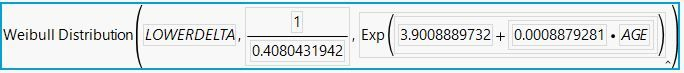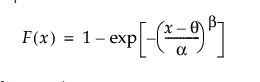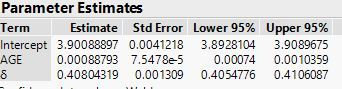Choose Language Hide Translation Bar
Highlighted

## Formula for Weibull distribution with a co-variate

Hello,

I performed a survival analysis with censored data, and a co-variate called "AGE". I used "Save Probabilty Formula" to save the formula in the data table. Now when  I right click the newly created column, and click on "Formula", I get the below formula.My question is could someone advise on the actual formula for Weibull Distribution where I can substitute the calibrated co-efficients, and input parameters to calculate the outputs?

I searched around on the JMP website and found the below formula for Weibull distribution:But I am not clear which co-efficient goes where, and what to do with the additional co-efficient of the co-variate "AGE". Below are the estimated parameters calibrated by JMP.I apologize, I am an engineer and I am not very stat-saavy.

Thank you,

Mike

2 REPLIES 2
Highlighted

## Re: Formula for Weibull distribution with a co-variate

I am not clear why you can't use the column formula to "calculate output."

There are two popular parameterizations of the Weibull model. JMP uses the location and scale version because it has better numerical properties when estimating the parameters. The more commonly used parameterization, though, is the shape and scale version. The formula you found is the latter version. It is easy to convert between them. In fact, the column formula has already done it for you.

The scale parameter alpha is equal to the reciprocal delta, so JMP's function shows the second argument as 1 / 0.4080431942. The shape parameter beta is equal to the log of mu, so JMP's function shows the third argument as e^(3.9008889732 + 0.0008879281 * Age). The shape parameter is a function of Age, while the scale parameter is independent of Age.

LOWERDELTA appears to be the quantile you used as a response. F(x) is the cumulative distribution function. It returns the probability of obtaining a value between 0 and the given quantile.

Learn it once, use it forever!
Highlighted

## Re: Formula for Weibull distribution with a co-variate

Hi Mark,

The reason why I want to know the formula and sort of "plugin" the parameters to evaluate the risk is I am trying to prepare a report by reporting the formula and associated parameters. So any one who is reading the report, irrespective of owning JMP, could "plugin" the parameters and the quantile to evaluate the risk.

Essentially, I will have to report the formula, and tabulate the respective parameters, which should enable the readers to evaluate risk for their chosen quantile (s).

Hope that makes sense.

Thanks,

Mike

Article Labels

There are no labels assigned to this post.